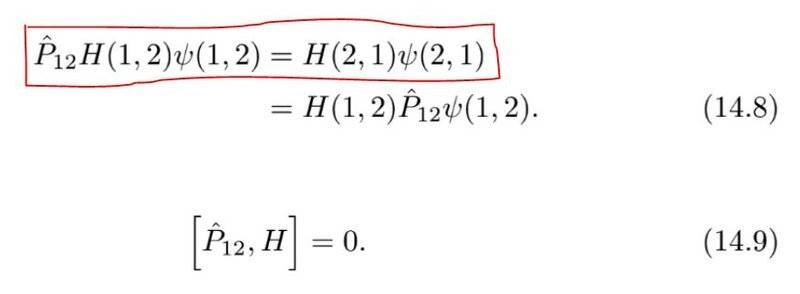# Deriving the Commutator of Exchange Operator and Hamiltonian

• I
• Samama Fahim

#### Samama FahimIn the boxed equation, how would you get the right hand side from the left hand side? We know that ##H(1,2) = H(2,1)##, but we first have to apply ##H(1,2)## to ##\psi(1,2)##, and then we would apply ##\hat{P}_{12}##; the result would not be ##H(2,1) \psi(2,1)##. ##\hat{P}_{12}## is the exchange operator and ##H(1,2)## is the hamiltonian.

Source: https://books.google.com.pk/books?i...inction as to which particle is which&f=false

Last edited:

$$P_{12}H(1,2)\psi(12) = P_{12}H(1,2){P_{12}}^\dagger P_{12}\psi(1,2) = H(2,1)\psi(2,1)$$
•